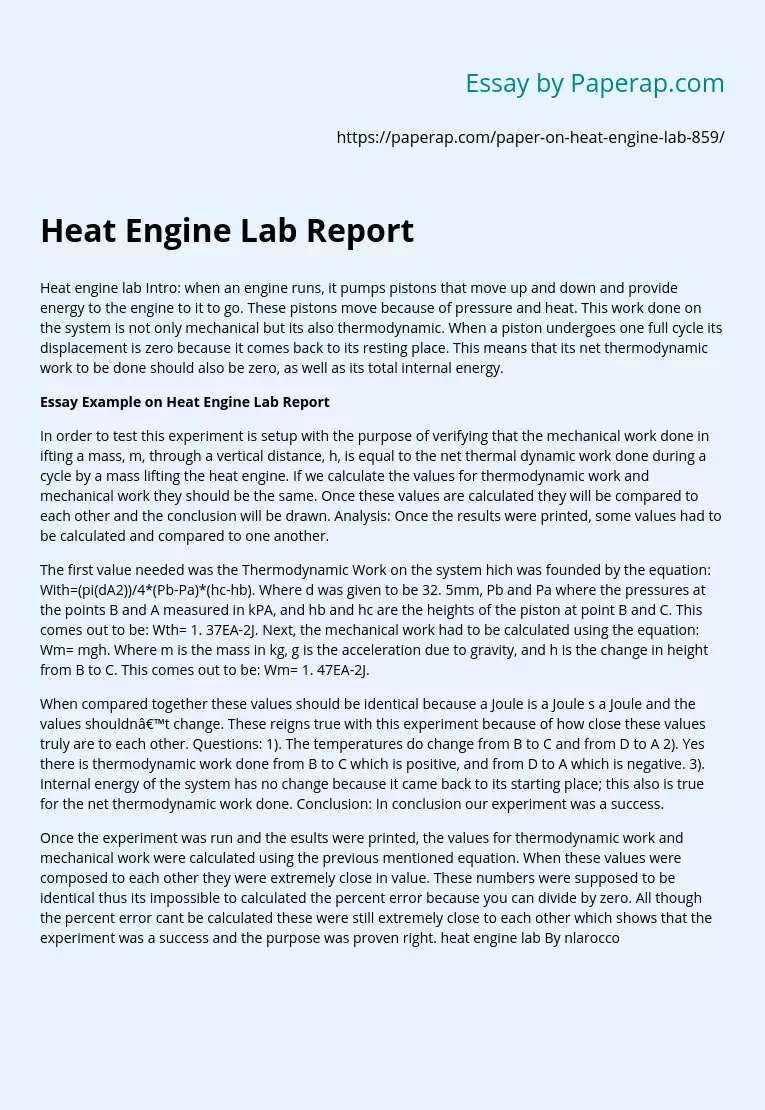# Heat Engine Lab Report

Heat engine lab Intro: when an engine runs, it pumps pistons that move up and down and provide energy to the engine to it to go. These pistons move because of pressure and heat. This work done on the system is not only mechanical but its also thermodynamic. When a piston undergoes one full cycle its displacement is zero because it comes back to its resting place. This means that its net thermodynamic work to be done should also be zero, as well as its total internal energy.

## Essay Example on Heat Engine Lab Report

In order to test this experiment is setup with the purpose of verifying that the mechanical work done in ifting a mass, m, through a vertical distance, h, is equal to the net thermal dynamic work done during a cycle by a mass lifting the heat engine. If we calculate the values for thermodynamic work and mechanical work they should be the same. Once these values are calculated they will be compared to each other and the conclusion will be drawn.

Analysis: Once the results were printed, some values had to be calculated and compared to one another.

The first value needed was the Thermodynamic Work on the system hich was founded by the equation: With=(pi(dA2))/4*(Pb-Pa)*(hc-hb). Where d was given to be 32. 5mm, Pb and Pa where the pressures at the points B and A measured in kPA, and hb and hc are the heights of the piston at point B and C.

Get quality help nowwriter-CharlotteVerified

Proficient in: Chemistry4.7 (348)

“ Amazing as always, gave her a week to finish a big assignment and came through way ahead of time. ”+84 relevant experts are online

This comes out to be: Wth= 1. 37EA-2J. Next, the mechanical work had to be calculated using the equation: Wm= mgh. Where m is the mass in kg, g is the acceleration due to gravity, and h is the change in height from B to C. This comes out to be: Wm= 1. 47EA-2J.

When compared together these values should be identical because a Joule is a Joule s a Joule and the values shouldn’t change. These reigns true with this experiment because of how close these values truly are to each other. Questions: 1). The temperatures do change from B to C and from D to A 2). Yes there is thermodynamic work done from B to C which is positive, and from D to A which is negative. 3). Internal energy of the system has no change because it came back to its starting place; this also is true for the net thermodynamic work done. Conclusion: In conclusion our experiment was a success.

Once the experiment was run and the esults were printed, the values for thermodynamic work and mechanical work were calculated using the previous mentioned equation. When these values were composed to each other they were extremely close in value. These numbers were supposed to be identical thus its impossible to calculated the percent error because you can divide by zero. All though the percent error cant be calculated these were still extremely close to each other which shows that the experiment was a success and the purpose was proven right. heat engine lab By nlarocco